# Complete lattice

(diff) ← Older revision | Latest revision (diff) | Newer revision → (diff)
A partially ordered set in which any subsethas a least upper bound and a greatest lower bound. These are usually called the join and the meet ofand are denoted by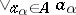andor simply by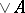and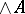(respectively). If a partially ordered set has a largest element and each non-empty subset of it has a greatest lower bound, then it is a complete lattice. A latticeis complete if and only if any isotone mappingof the lattice into itself has a fixed point, i.e. an element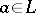such that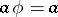. If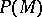is the set of subsets of a setordered by inclusion andis a closure operation on, then the set of all-closed subsets is a complete lattice. Any partially ordered setcan be isomorphically imbedded in a complete lattice, which in that case is called a completion of. The completion by sections (cf. Completion, MacNeille (of a partially ordered set)) is the least of all completions of a given partially ordered set. Complete lattices are formed by the set of all subalgebras in a universal algebra, by the set of all congruences in a universal algebra, and by the set of all closed subsets in a topological space.